Courses

# Ex 1.4 NCERT Solutions - Number System Class 9 Notes | EduRev

## Class 9 Mathematics by Full Circle

Created by: Full Circle

## Class 9 : Ex 1.4 NCERT Solutions - Number System Class 9 Notes | EduRev

The document Ex 1.4 NCERT Solutions - Number System Class 9 Notes | EduRev is a part of the Class 9 Course Class 9 Mathematics by Full Circle.
All you need of Class 9 at this link: Class 9

Ques 1: Visualise 3.765 on the number line, using successive magnification.
Ans:
3.765 lies between 3 and 4.
Let us divide the interval (3, 4) into 10 equal parts.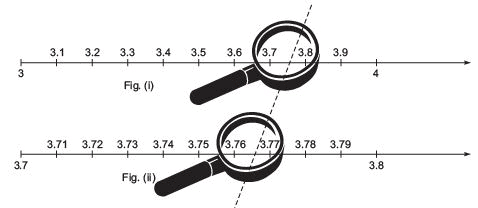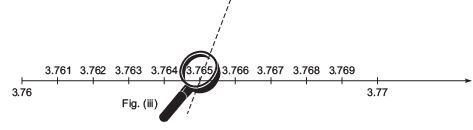Since, 3.765 lies between 3.7 and 3.8. We again magnify the interval [3.7, 3.8] by dividing it further into 10 parts and concentrate the distance between 3.76 and 3.77.
The number 3.765 lies between 3.76 and 3.77. Therefore we further magnify the interval [3.76, 3.77] into 10 equal parts.
Now, the point corresponding to 3.765 is clearly located, as shown in Fig. (iii) above.
Ques 2: Visualise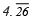on the number line, up to 4 decimal places.
Ans: Note:
We can magnify an interval endlessly using successive magnification.
To visualizeor 4.2626… on the number line up to 4 decimal places, we use the following steps.

I . The number 4.2626… lies between 4 and 5. Divide the interval [4, 5] in 10 smaller parts: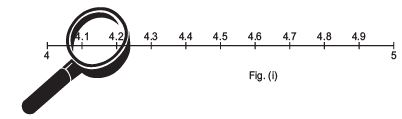II. Obviously, the number 4.2626... lies between 4.2 and 4.3. We magnify the interval [4.2, 4.3]: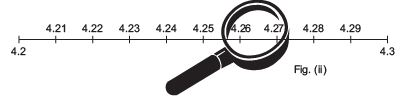III. Next, we magnify the interval [4.26, 4.27]: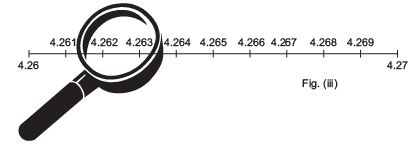IV. Finally magnify the interval [4.262, 4.263]: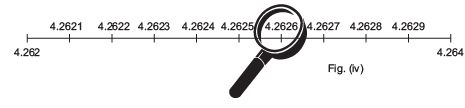In Fig. (iv), we can easily observe the number 4.2626… or.

,

,

,

,

,

,

,

,

,

,

,

,

,

,

,

,

,

,

,

,

,

;